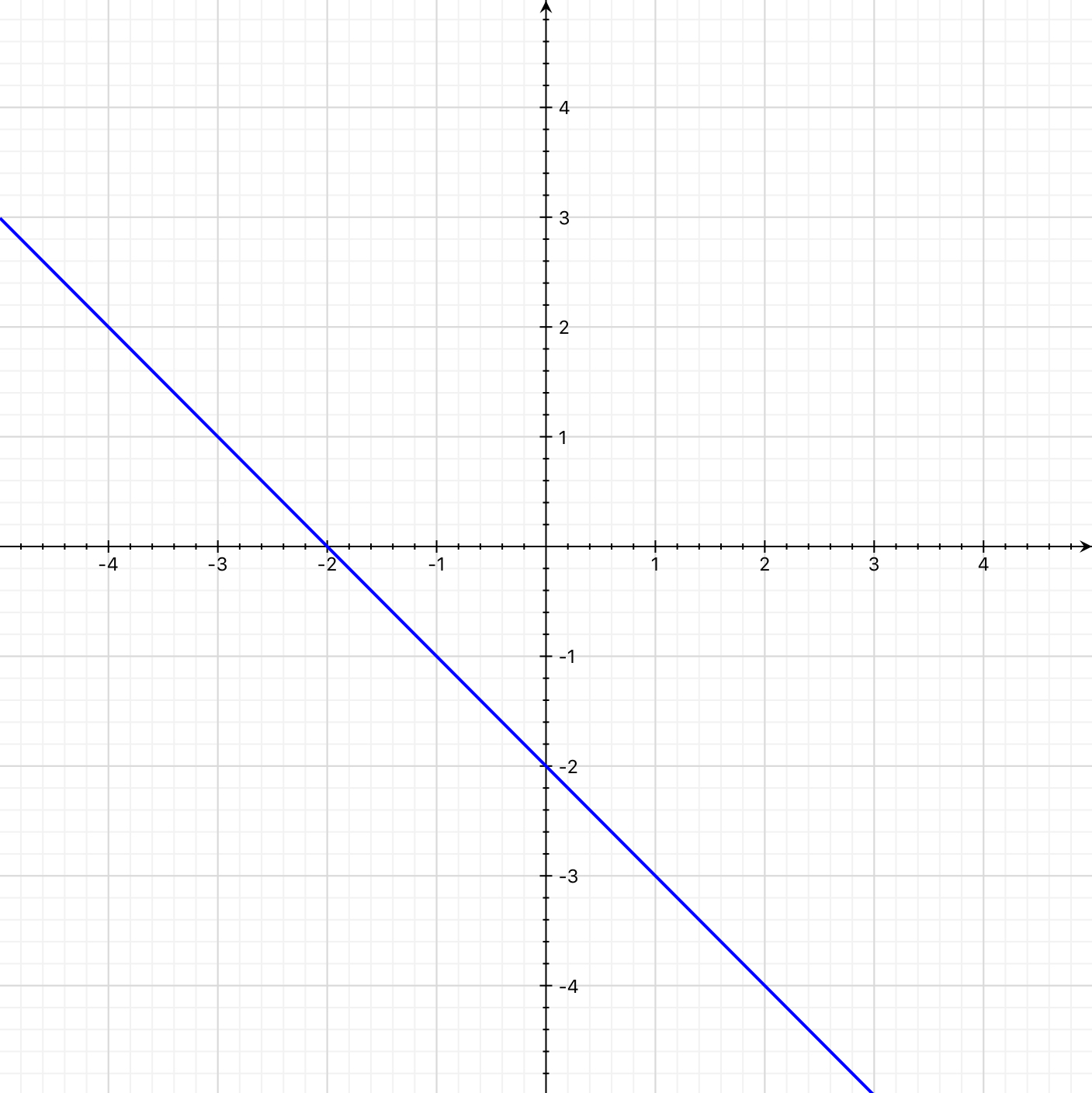## Finding a Line’s Equation from the Graph of Its PerpendicularThis is the line whose perpendicular you need to find.

This post about finding the equation of a line that is perpendicular to a line you read from a graph is part of a series of posts to help you prepare for the Advanced Algebra and Functions part of the Accuplacer test.

## Question

Find the equation of the line that goes through the origin and is perpendicular to the blue line shown.

## Solution

The origin is the point on the coordinate plane with the coordinates .

You are asked to find the equation of the line that is perpendicular to the one shown and passes through the origin.

### Slope

How do you find the equation of a perpendicular line? Start with the slope: If two lines are perpendicular and their slopes are    and  , then . One is the negative reciprocal of the other. Another way to say this: .

Next, find  , the slope of the line shown. Then the slope of the line whose equation we seek equals .

To do that, choose two points with coordinates you can read. The intercepts — (-2, 0) and (0, -2) — suggest themselves. The slope of the line between those points is the difference between their y-values divided by the difference between their x-values. The difference in y-values is . The difference in x-values is . Then the slope of the line between the points is:

So the original line has a slope . Then the line we are looking for has a slope  .

### Intercept

From there, all we need for the line’s equation is the y-intercept. You’re told the line passes through the point (0, 0), so the y-intercept — the b in  — is 0.

A line that passes through the origin has a y-intercept of 0.

Then this line’s y-intercept is 0 and its equation is

That’s the brown line on the graph below.The brown line is a perpendicular that passes through the origin.

The brown line looks perpendicular to the blue one and goes through the origin. Looks about right.

For more about relating a line’s equation to its graph, see “Comparing Data in Different Formats.

This question is similar to question number 2 in the sample questions for the Accuplacer Advanced Algebra and Functions test.